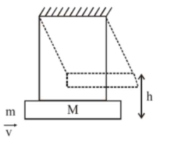# A large block of wood of massQuestion:

A large block of wood of mass $\mathrm{M}=5.99 \mathrm{~kg}$ is hanging from two long massless cords. A bullet of mass $\mathrm{m}=10 \mathrm{~g}$ is fired into the block and gets embedded in it. The (block + bullet) then swing upwards, their centre of mass rising a vertical distance $\mathrm{h}=9.8 \mathrm{~cm}$ before the (block $+$ bullet) pendulum comes momentarily to rest at the end of its arc. The speed of the bullet just before collision is : (Take $\mathrm{g}=9.8 \mathrm{~ms}^{-2}$ )1. (1) $841.4 \mathrm{~m} / \mathrm{s}$

2. (2) $811.4 \mathrm{~m} / \mathrm{s}$

3. (3) $831.4 \mathrm{~m} / \mathrm{s}$

4. (4) $821.4 \mathrm{~m} / \mathrm{s}$

Correct Option: , 3

Solution:

(3)

From energy conservation,

[ after bullet gets embedded till the system comes momentarily at rest

$(\mathrm{M}+\mathrm{m}) \mathrm{gh}=\frac{1}{2}(\mathrm{M}+\mathrm{m}) \mathrm{v}_{1}^{2}$

$\left[\mathrm{v}_{1}\right.$ is velocity after collision $]$

$\therefore \mathrm{v}_{1}=\sqrt{2 \mathrm{gh}}$

Applying momentum conservation, (just

before and just after collision)

$\mathrm{mv}=(\mathrm{M}+\mathrm{m}) \mathrm{v}_{1}$

$\mathrm{v}=\left(\frac{\mathrm{M}+\mathrm{m}}{\mathrm{m}}\right) \mathrm{v}_{1}=\frac{6}{10 \times 10^{-3}} \times \sqrt{2 \times 9.8 \times 9.8 \times 10^{-2}}$

$\approx 831.55 \mathrm{~m} / \mathrm{s}$